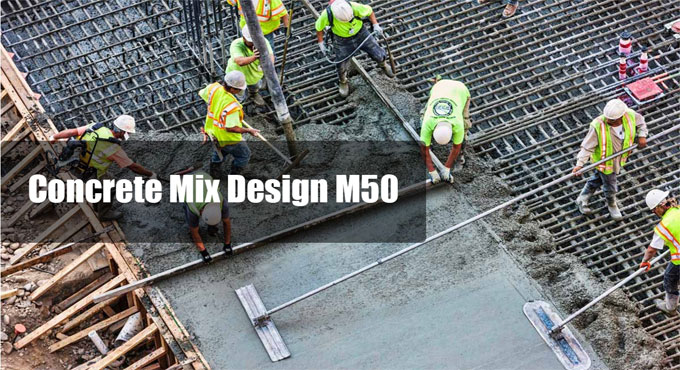# Specification of M50 concrete mix designConcrete mix design belongs to the method of determining the ratios of concrete mix in terms of proportions of cement, sand and coarse aggregates.

As for instance a concrete mix of proportions 1:2:4 signifies that cement, fine and coarse aggregate are in the ratio 1:2:4 or the mix comprises of one part of cement, two parts of fine aggregate and four parts of coarse aggregate.

The concrete mix design proportions are both by volume or by mass. The water-cement ratio is normally stated in mass.

The mix design M-50 grade (with Admixture –Sikament) presented here is for reference purpose only. Actual site conditions may fluctuate and consequently, this should be modified as per the location and other factors.

Given below the specifications of M50 Concrete Mix Design

Type of cement = O.P.C-43 grade
Brand of cement = Vikram ( Grasim )
Admixture = Sika [Sikament 170 ( H ) ]
Fine Aggregate = Zone-II
Sp. Gravity
Cement = 3.15

Fine Aggregate = 2.61
Coarse Aggregate (20mm) = 2.65
Coarse Aggregate (10mm) = 2.66
Minimum Cement (As per contract) =400 kg / m3
Maximum water cement ratio (As per contract) = 0.45

Mix Calculation: –
1. Target Mean Strength = 50 + ( 5 X 1.65 ) = 58.25 Mpa

2. Selection of water cement ratio:-
Suppose water cement ratio = 0.35

3. Calculation of water: Estimated water content for 20mm max. Size of aggregate = 180 kg /m3 (As per Table No. 5 , IS : 10262 ). As plasticizer is projected, water content is decreased by 20%.

Water content is taken as 180 X 0.8 = 144 kg /m3

For more details, click on the following link engineeringcivil.com